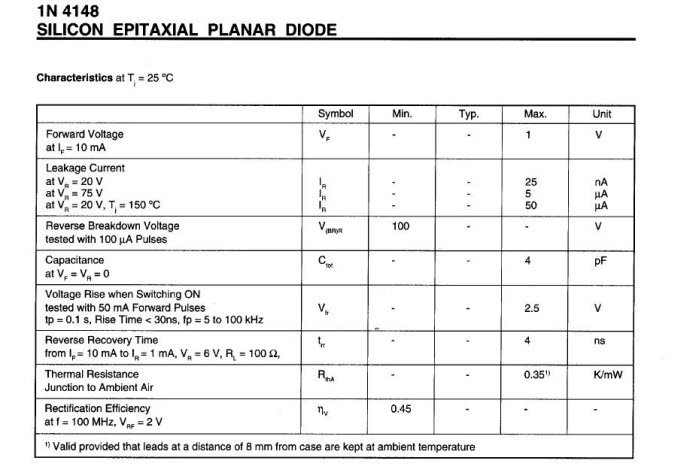# SPICE modeling of a Diode from Datasheet

Modeling in SPICE& a diode is not a trivial work. Although the operation of the diode is quite simple, extract a model from datasheet takes some time.Every component has its own syntax defined in SPICE , in the case of the diode:

.model ModelName D (par1=a par2=b………parn=x)

where par1 par2 …. parn are characteristic parameters of diode.

we can sum up the set of main parameters in the following table:

ParameterDescriptionUnitDefault value
BVReverse breakdown knee voltageVInfinite
CJOZero-bias p-n capacitanceF0
EGBandgap voltageeV1.11
FCForward-bias depletion capacitance coefficientno unit dimension0.5
IBLVLow-level reverse breakdown knee correntA0
IBVReverse breakdown knee correntA1e-10
IKFHigh-injection knee currentAInfinite
ISSaturation correntA1e-14
ISRRecombination current parameterA0
NEmission coefficientno unit dimension1.0
NREmission coefficient for ISRno unit dimension2.0
RSParasitic resistanceOhm0
TTTransit timesec0
VJp-n potentialV1.0
XTIIS temperature exponentno unit dimension3.0

All these parameters are used by SPICE to describe the behavior of the diode in the different situations of signal, for example in direct polarization in DC that, forward current will be:

ID = IS*(e^(VD/(N*Vt))-1)

where VD is the forward voltage, Vt = k * T / q is the thermal voltage equal to 0.026 V at 27 degrees Celsius.

The so-called recombination current is instead calculated as

Irec = ISR*(e^(VD/(N*Vt))-1).

Other equations from the given parameters describing the capacitance of the junction, its evolution with temperature and more.

At this point we have to derive the various parameters from the datasheet of the component. Assume we want to model a silicon diode 1N4148. The extraction of the parameters of the table from the values reported in the datasheet, is not immediate for almost none of the parameters.look at the values of our interest in datasheet:From the table we can get BV which is equal to VRM, in other cases reported as Vbr, or in the case of Zener diode Vz.From this second table we see that the maximum leakage current at 25 degrees is Ir = 5 uA.We can take IBV as equal to 10 times Ir. Usually for this type of diodes the value of IBV is around 100uA. For Zener diodes Ir can be called Izk, or in other cases as Ibr.

CJO can be directly equal to the value specified in the datasheet as Cj or Ctot, in this case is 4pF.

Posted in PSpice Modeling from Datasheet and tagged , , , .